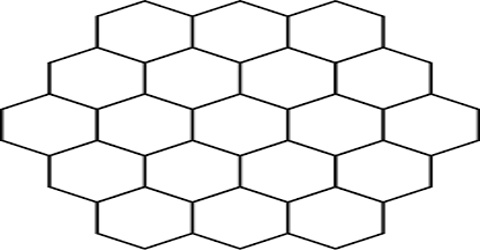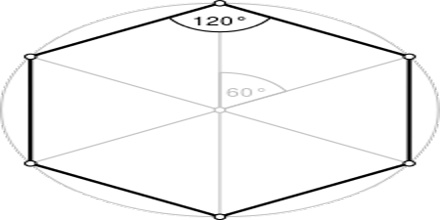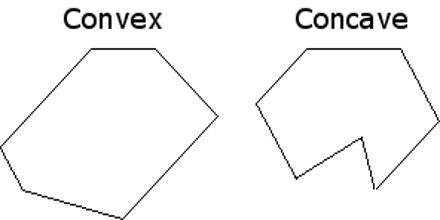Mathematic

# Hexagon Polygon: Overview with TypesHexagon is a six-sided polygon (a flat shape with straight sides). It is a polygon of six angles and six sides. The total of the internal angles of any hexagon is 720°. A hexagon does have 6 vertices, 6 interior angles and 6 sides.

Hexagons are found in many other parts of nature: the bond-shapes of certain molecules, in crystal structures, in the patterns of turtle shells, and more.

Properties

A regular hexagon has:

• Interior Angles of 120°
• Exterior Angles of 60°
• Area = (1.5√3) × s2, or approximately 2.5980762 × s2 (where s = side length)Types of Hexagon:

(1) Regular Hexagon: A regular hexagon has all sides equal in length and it also has all angles equal. The measure of each interior angle of a regular hexagon is: 720°/6 or 1200.

(2) Irregular Hexagon: A hexagon that does not have all sides equal, is known as an irregular hexagon. It does not have all angles same. In other words, a hexagon that is not regular, is categorized as an irregular hexagon.(3) Concave Hexagon: A concave hexagon is a type of hexagon which has at least one interior angle measuring greater than 180∘180∘. This hexagon has at least a single vertex that points inwards. It can be a regular concave or an irregular concave hexagon.

(4) Convex Hexagon: A hexagon that has all the interior angles less than 180∘180∘ is called a convex hexagon. It has all its vertices pointing outward. This type of hexagon is very often used in geometry and its application.